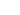# GOOGLESHEET Function

This article explains how to use GOOGLESHEET functions

# Sample Usage

``GOOGLESHEET("1qYJVonS_caYCc1diSOYb0HM-s5nUyxW6PsfsvzIoJK9I", "2050638375")``

# Syntax

``GOOGLESHEET(spreadsheet_id, sheet_id)``
• `spreadsheet_id` - The ID `string` of the Google spreadsheet, that can be found in the URL of the spreadsheet
• `sheet_id` - The ID `string` of the sheet (or sometimes called worksheet), that can be found in teh URL of the spreadsheet.
• Before you can use the GOOGLESHEET function you need to connect your Google account. You can set connect your account in the connections section of the account page.
• The return value from this function is a matrix (or table like dataset) with rows and columns.
• You can find the `spreadsheet_id` and `sheet_id` in the URL of your google spreadsheet. Open your spreadsheet and find the `spreadsheet_id` and `sheet_id` here:# Examples

You can use the data from the GOOGLESHEET function exactly like how you would use data from datasheets. Here are some examples:

## Returning a single value from the matrix

If you want to return a single value, you can do so in conjunction with the `INDEX` function:

``INDEX(GOOGLESHEET("spreadsheet_id", "sheet_id"), 0, 0)``

This returns the value in the cell of the first row of the first column. You can use this together with other inputs (like questions or variables) to make it a bit more interesting:

``INDEX(GOOGLESHEET("spreadsheet_id", "sheet_id"), QA, QB)``

Now the value is returned based on the outcome of QA and QB.

## Using VLOOKUP or HLOOKUP

Using VLOOKUP or HLOOKUP works exactly the same as with datasheets:

``VLOOKUP(123, GOOGLESHEET("spreadsheet_id", "sheet_id"), 1, 0)``

This will find the row where 123 is the first column and returns the second column. Again, let's make it more interesting:

``VLOOKUP(QA{text}, GOOGLESHEET("spreadsheet_id", "sheet_id"), QB, 1)``

Now we're talking! If a users fills out a text field, it searches all the rows of the first column of your spreadsheet to find a partial match. Depending on the value of QB it returns that column.

## Using SUMIF, SUMIFS, FINDIF, FINDIFS

Using SUMIF, SUMIFS, and FINDIFS is possible as well. It works a little bit differently when using GOOGLESHEET() compared to datasheets:

### Datasheet

``SUMIFS(DA_C, DA_A, QA, DA_B, QB)``

### GOOGLESHEET()

``````VA = GOOGLESHEET("spreadsheet_id", "sheet_id")

FINDIFS(COLUMN(VA, 2), COLUMN(VA, 0), QA, COLUMN(VA, 1), QB)``````

In this example we first created a variable (`VA`) for getting the spreadsheet data. then for FINDIFS we need to reference the columns A, B, and C a little bit diffently:

• DA_C = COLUMN(VA, 2)
• DA_A = COLUMN(VA, 0)
• DA_B = COLUMN(VA, 1)

Note that counting starts at 0, so the first column (A) is refered to by 0, the second column by 1 etc.

This works the same for `SUMIF` and `SUMIFS`.